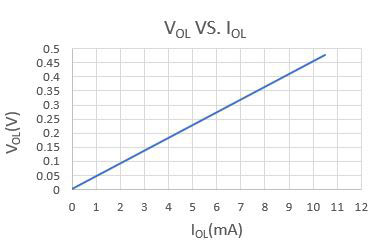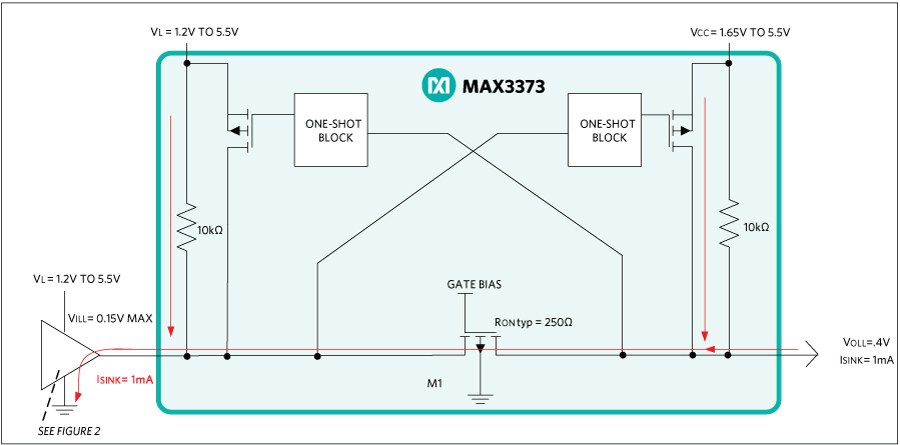# Explaining the Vill Logic Level Specification for MAX3373 Logic Level Translator

### Abstract

Logic-level specifications are simple. Yet, occasionally, there is a logic threshold specification that appears ambiguous. Electronics usually has an explanation for most ambiguities. Occasional questions also arise on the VILL specification for the logic-level translator and lower maximum value. This application note explains the origin of this specification.

### Introduction

The VILL specification of 0.15V is a very common question about the logic-level translator. The common logic specifications for VOL (for a logic output) are customarily specified at the different current sinking levels. They are higher than 0.15V. This usually is confusing. This application note explains the origin of these specifications. Figure 1 is a snippet of the VOL specification from a GPIO (general-purpose input/output) port of the MAX32600 low-power MCU (microcontroller unit). Similar specifications are found in the FPGA (field programmable gate arrays), CPLD (complex programmable logic devices), and other logic device data sheets.

 Output Low Voltage for All Port Pins VOL VDD = 3.6V, IOL = 11mA 0.4 0.5 V VDD = 2.3V, IOL = 8mA 0.4 0.5

Figure 1. VOL Specification from the MAX32600 Low-Power MCU.

The typical output structure for the CMOS (complementary metal-oxide semiconductor) logic has a high-side Q1 PCH transistor and low-side Q2 NCH transistor (Figure 2). The output Q2 is turned on for the logic-low. The output Q1 is turned on and Q2 turned off for the logic-high. There is a direct correlation of the RON (on-resistance) of Q2 for the VOL based on the specified IOL current from the condition column. The maximum VOL is 0.5V when IOL = 11mA with VDD = 3.6V (Figure 1). The RON for Q2 is then 0.5V/.011A or 45.45O. Figure 3 provides a good breakdown of the expected VOL values versus the IOL current. The actual logic-low voltage must be 0.045V for IOL = 1mA. This is well below the VIL of 0.15V listed in the MAX3373 data sheet.Figure 2. Typical Output Structure of the CMOS Logic.Figure 3. Logic-Low Level (VOL) vs. Sink Current (IOL).

### Logic-Level Specifications

See the logic-level specifications for VIL, VIH, VOL, and VOH (Figure 4), and how they relate to the topology of the level translator.

The following are the input and output logic-level specifications from the MAX3373 data sheet.

Logic-Level Thresholds (MAX3373E–MAX3376E/MAX3378E/MAX3379E and MAX3390E–MAX3393E)
I/O VL_ Input-Voltage High VIHL VL - 0.2 V
I/O VL_ Input-Voltage Low VILL 0.15

V

I/O VCC_ Input-Voltage High VIHC VCC - 0.4 V
I/O VCC_ Input-Voltage Low VILC 0.15 V
I/O VL_ Output-Voltage High VOHL I/O VL_ source current = 20µA,
I/O VCC_ = VCC - 0.4V
0.67 x VL V
I/O VL_ Output-Voltage Low VOLL I/O VL_ sink current = 1mA,
I/O VCC_ = 0.15V
0.4 V
I/O VCC_ Output-Voltage High VOHC I/O VCC_ source current = 20µA,
I/O VL_ = VL - 0.2V
0.67 x VCC V
I/O VCC_ Output-Voltage Low VOLC I/O VCC_ sink current = 1mA,
I/O VL_ = 0.15V
0.4 V
Three-State Input-Voltage High VIH-Three-State VL - 0.2 V
Three-State Input-Voltage Low VIL-Three-State 0.15 V
Figure 4. Logic-Level Thresholds.

The translator input of 150mV is the most common confusion when interpreting the VILL specification.

### The Origin of the Specification

The VOLL specification (Figure 4) is a maximum of 0.4V upon closer inspection. This specification is governed by the condition column, which shows the I/O sink current = 1mA I/O = 0.15V. The conditions are the same for both I/O VL and I/O VCC. See the simplified circuit structure in Figure 5 to understand these specifications.The MOS Transistor M1 acts as a closed switch with some resistance when the logic input is low. The MOS Transistor M1 is off and prevents the flow of current when the logic input is high.

The VILL specification has a maximum value of 0.150V and VOLL a maximum value of 0.400V when the sinking value is 1mA. Thus, there may be an external pullup on the output side of the translator. The approximate resistance of the MOS transistor when biased on is:

RON = (0.4V - 0.15V)/1mA = 250?

For example, using the circuit depicted in Figure 5, if VCC = 3.3V, the nominal sink current from the internal 10k? pullup is 3.3V - 0.4V/10K = 290µA. The actual logic input can be as high as 0.4V - (290uA x 250O) = 0.327V instead of 0.15V for VILL (max) if the VOLL is set to a maximum of 0.4V using the RON value of 250O with no external pullup.

### Summary

The MAX3373 VILL specification of 0.15V seems hard to achieve immediately. One must closely look at the sink current from the logic output driving the input of the level translator. The specifications for the logic output of the MCUs, FPGAs, etc., are based on the current sinking levels much higher than 1ma. The VOL is much lower when interfacing with Maxim's logic level translator. For example, the maximum VOL is specified at 0.5V when the sinking level is 11mA (Figure 1). This implies that the low-side switch element for the logic gate has a resistance of 0.5V/11mA = 45O. The logic-low output is 0.045V at 1mA, which is well below the 0.15V requirement.